#Function Repository Resource:

# CscDegree

Compute the cosecant of an angle given in degrees

Contributed by: Paco Jain (Wolfram Research)
 ResourceFunction["CscDegree"][x] computes the cosecant of an angle x, measured in degrees.

## Details and Options

Mathematical function, suitable for both symbolic and numerical manipulation.
For certain special arguments, CscDegree automatically evaluates to exact values.
CscDegree can be evaluated to arbitrary numerical precision.

## Examples

### Basic Examples

Compute the cosecant of an angle:

 In:=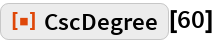Out=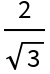Plot over a given range of angles:

 In:=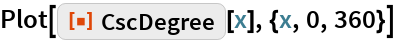Out=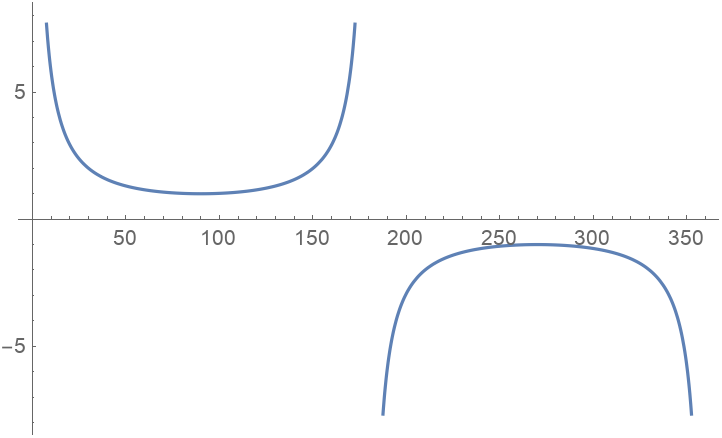### Scope

Evaluate numerically:

 In:=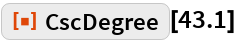Out=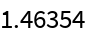Evaluate to high precision:

 In:=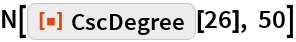Out=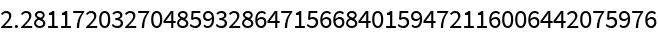## Requirements

Wolfram Language 11.3 (March 2018) or above